Maths-
General
Easy

Question

# The diameter of a metallic ball is 4.2 cm. What is the mass of the ball , if the density of the metal is 8.9 g per cubic cm ?Hint:

## The correct answer is: = 2762.5511gm

### Step 1 of 1:We will first find the volume of the metallic ball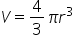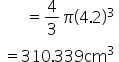Now we know thatDensity =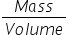Put the value of density and volume in formula, we will get massDensity =8.9 =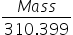Mass = 8.9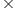310.399gm= 2762.5511gm#### With Turito Foundation.#### Get an Expert Advice From Turito.